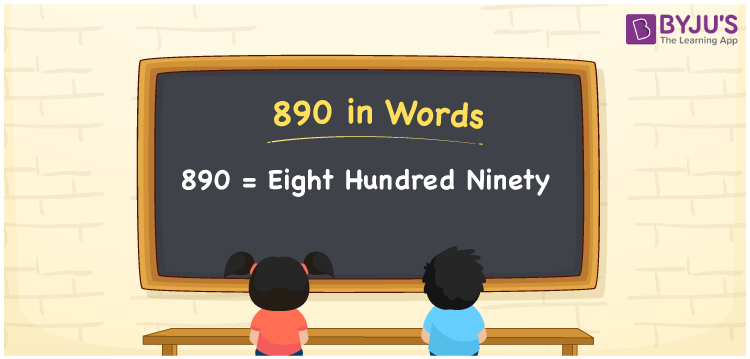# 890 in words

890 in words is written as Eight Hundred and Ninety. 890 represents the count or value. The article on Counting Numbers can give you an idea about count or counting. The number 890 is a 3 digit number that is used in expressions related to money, days, distance, length, weight and so on. Let us consider an example for 890. “A theater group has performed Eight Hundred and Ninety shows.”

 890 in words Eight Hundred and Ninety Eight Hundred and Ninety in Numbers 890

## 890 in English Words## How to Write 890 in Words?

We can convert 890 to words using a place value chart. The number 890 has 3 digits, so let’s make a chart that shows the place value up to 3 digits.

 Hundreds Tens Ones 8 9 0

Thus, we can write the expanded form as:

8 × Hundred + 9 × Ten + 0 × One

= 8 × 100 + 9 × 10 + 0 × 1

= 890

= Eight Hundred and Ninety.

890 is the natural number that is succeeded by 889 and preceded by 891.

890 in words – Eight Hundred and Ninety.

Is 890 an odd number? – No.

Is 890 an even number? – Yes.

Is 890 a perfect square number? – No.

Is 890 a perfect cube number? – No.

Is 890 a prime number? – No.

Is 890 a composite number? – Yes.

## Solved Example

1. Write the number 890 in expanded form

Solution: 8 × 100 + 9 × 10 + 0 × 1

We can write 890 = 800 + 90 + 0

= 8 × 100 + 9 × 10 + 0 × 1.

## Frequently Asked Questions on 890 in words

Q1

### How to write the number 890 in words?

890 in words is written as Eight Hundred and Ninety.
Q2

### Is 890 divisible by 3?

No. 890 is not divisible by 3.
Q3

### Is 890 a prime number?

No. 890 is not a prime number.